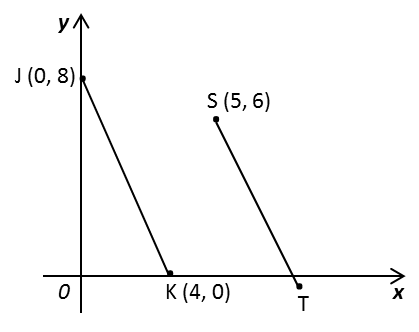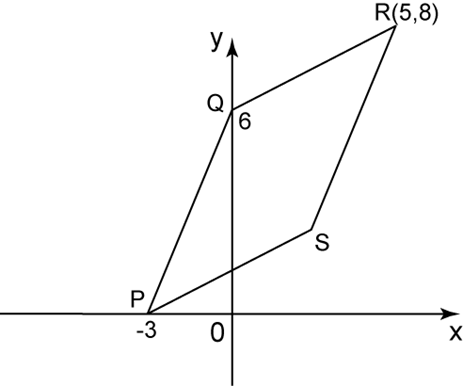# 9.3.2 Straight Lines, PT3 Focus Practice

Question 3:
Diagram below shows a straight line JK and a straight line ST drawn on a Cartesian plane. JK is parallel to ST.Find
(a) the equation of the straight ST,
(b) the x-intercept of the straight line ST.

Solution:
(a)
JK is parallel to ST, therefore gradient of JK = gradient of ST.
$\begin{array}{l}=\frac{8-0}{0-4}\\ =-2\end{array}$
Substitute m = –2 and S (5, 6) into y = mx + c
6 = –2 (5) + c
c = 16
Therefore equation of ST: y = –2x + 16

(b)

For x-intercept, y = 0
0 = –2x + 16
2x = 16
x = 8
Therefore x-intercept of ST = 8

Question 4:In the diagram above, PQRS is a parallelogram. Find
$\begin{array}{l}\text{For x-intercept,}y=0\\ 0=\frac{2}{5}x+6\\ x=-15\end{array}$